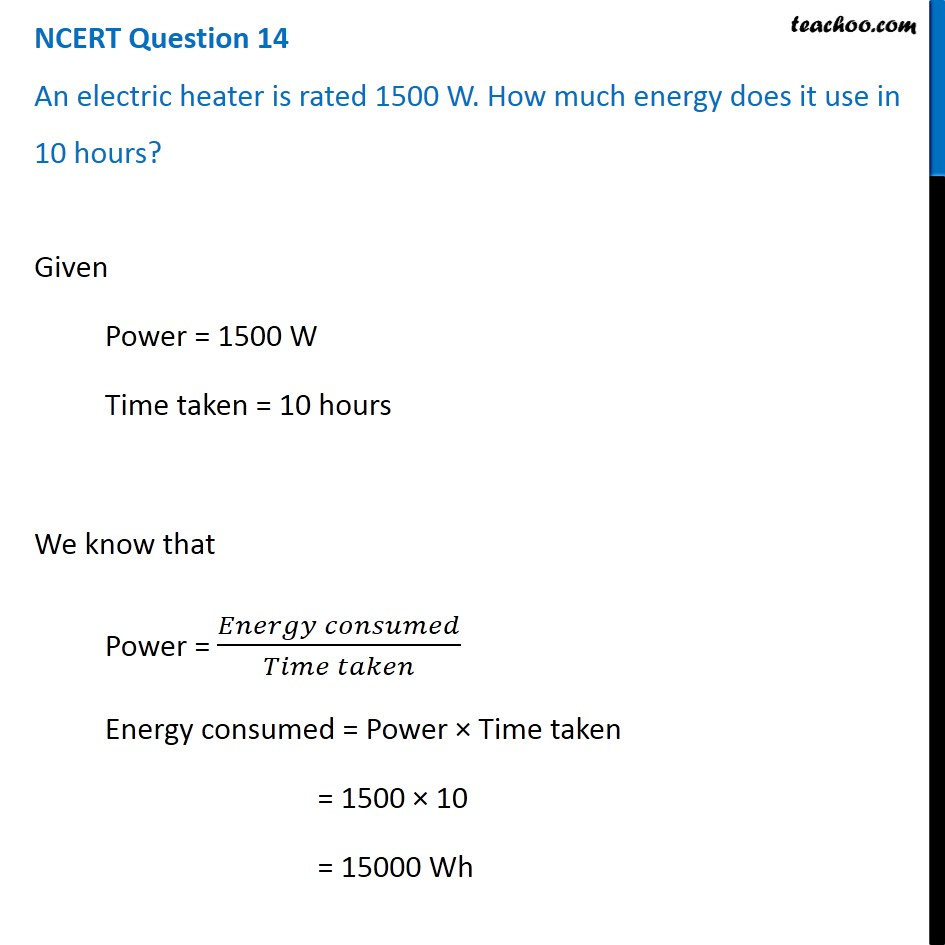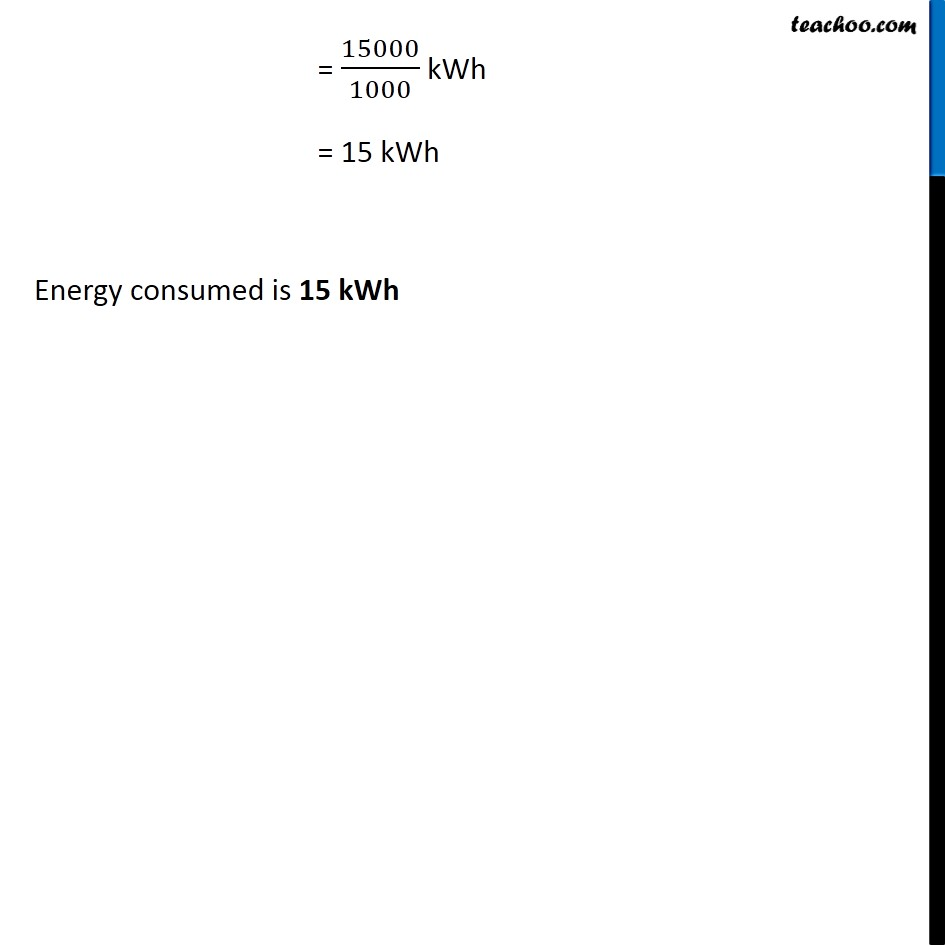1. Class 9
2. Chapter 11 Class 9 - Work and Energy (Term 2)
3. NCERT Questions

Transcript

NCERT Question 14 An electric heater is rated 1500 W. How much energy does it use in 10 hours? Given Power = 1500 W Time taken = 10 hours We know that Power = (𝐸𝑛𝑒𝑟𝑔𝑦 𝑐𝑜𝑛𝑠𝑢𝑚𝑒𝑑)/(𝑇𝑖𝑚𝑒 𝑡𝑎𝑘𝑒𝑛) Energy consumed = Power × Time taken = 1500 × 10 = 15000 Wh = 15000/1000 kWh = 15 kWh Energy consumed is 15 kWh

NCERT Questions

Class 9
Chapter 11 Class 9 - Work and Energy (Term 2)